Test: DFT Properties

# Test: DFT Properties

Test Description

## 10 Questions MCQ Test Signals and Systems | Test: DFT Properties

Test: DFT Properties for Electrical Engineering (EE) 2023 is part of Signals and Systems preparation. The Test: DFT Properties questions and answers have been prepared according to the Electrical Engineering (EE) exam syllabus.The Test: DFT Properties MCQs are made for Electrical Engineering (EE) 2023 Exam. Find important definitions, questions, notes, meanings, examples, exercises, MCQs and online tests for Test: DFT Properties below.
Solutions of Test: DFT Properties questions in English are available as part of our Signals and Systems for Electrical Engineering (EE) & Test: DFT Properties solutions in Hindi for Signals and Systems course. Download more important topics, notes, lectures and mock test series for Electrical Engineering (EE) Exam by signing up for free. Attempt Test: DFT Properties | 10 questions in 10 minutes | Mock test for Electrical Engineering (EE) preparation | Free important questions MCQ to study Signals and Systems for Electrical Engineering (EE) Exam | Download free PDF with solutions
 1 Crore+ students have signed up on EduRev. Have you?
Test: DFT Properties - Question 1

### If X(k) is the N-point DFT of a sequence x(n), then what is the DFT of x*(n)?

Detailed Solution for Test: DFT Properties - Question 1

According to the complex conjugate property of DFT, we have if X(k) is the N-point DFT of a sequence x(n), then what is the DFT of x*(n) is X*(N-k).

Test: DFT Properties - Question 2

### If X(k) is the N-point DFT of a sequence x(n), then circular time shift property is that N-point DFT of x((n-l))N is X(k)e-j2πkl/N.

Detailed Solution for Test: DFT Properties - Question 2

Explanation: According to the circular time shift property of a sequence, If X(k) is the N-point DFT of a sequence x(n), then the N-pint DFT of x((n-l))N is X(k)e-j2πkl/N.

Test: DFT Properties - Question 3

### What is the circular convolution of the sequences x1(n)={2,1,2,1} and x2(n)={1,2,3,4}, find using the DFT and IDFT concepts?

Detailed Solution for Test: DFT Properties - Question 3

Explanation: Given x1(n)={2,1,2,1}=>X1(k)=[6,0,2,0] Given x2(n)={1,2,3,4}=>X2(k)=[10,-2+j2,-2,-2-j2] when we multiply both DFTs we obtain the product
X(k)=X1(k).X2(k)=[60,0,-4,0] By applying the IDFT to the above sequence, we get
x(n)={14,16,14,16}.

Test: DFT Properties - Question 4

What is the circular convolution of the sequences x1(n)={2,1,2,1} and x2(n)={1,2,3,4}?

Detailed Solution for Test: DFT Properties - Question 4

Explanation: We know that the circular convolution of two sequences is given by the expression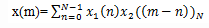For m=0,x2((-n))4={1,4,3,2}
For m=1,x2((1-n))4={2,1,4,3}
For m=2,x2((2-n))4={3,2,1,4}
For m=3,x2((3-n))4={4,3,2,1}
Now we get x(m)={14,16,14,16}.

Test: DFT Properties - Question 5

If x1(n),x2(n) and x3(m) are three sequences each of length N whose DFTs are given as X1(k),X2(k) and X3(k) respectively and X3(k)=X1(k).X2(k), then what is the expression for x3(m)?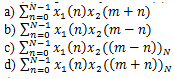Detailed Solution for Test: DFT Properties - Question 5

Explanation: If x1(n),x2(n) and x3(m) are three sequences each of length N whose DFTs are given as X1(k),X2(k) and X3(k) respectively and X3(k)=X1(k).X2(k), then according to the multiplication property of DFT we have x3(m) is the circular convolution of x1(n) and x2(n).

Test: DFT Properties - Question 6

. If x(n) is real and odd, then what is the IDFT of the given sequence?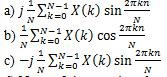d) None of the mentioned

Detailed Solution for Test: DFT Properties - Question 6

Explanation: If x(n) is real and odd, that is x(n)=-x(N-n), then XR(k)=0. Hence X(k) is purely imaginary and odd. Since XR(k) reduces to zero, the IDFT reduces to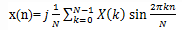Test: DFT Properties - Question 7

If x(n) is real and even, then what is the DFT of x(n)?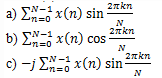d) None of the mentioned

Detailed Solution for Test: DFT Properties - Question 7

Explanation: Given x(n) is real and even, that is x(n)=x(N-n)
We know that XI(k)=0. Hence the DFT reduces to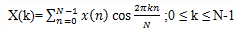Test: DFT Properties - Question 8

If x(n) is a real sequence and X(k) is its N-point DFT, then which of the following is true?

Detailed Solution for Test: DFT Properties - Question 8

Explanation: We know that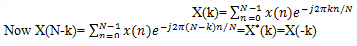Therefore,
X(N-k)=X*(k)=X(-k)

Test: DFT Properties - Question 9

If x(n) is a complex valued sequence given by x(n)=xR(n)+jxI(n), then what is the DFT of xR(n)?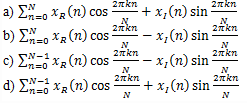Detailed Solution for Test: DFT Properties - Question 9

Explanation: Given x(n)=xR(n)+jxI(n)=> xR(n)=1/2(x(n)+x*(n))
Substitute the above equation in the DFT expression
Thus we get,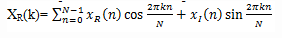Test: DFT Properties - Question 10

If X1(k) and X2(k) are the N-point DFTs of x1(n) and x2(n) respectively, then what is the N-point DFT of x(n)=ax1(n)+bx2(n)?

Detailed Solution for Test: DFT Properties - Question 10

Explanation: We know that, the DFT of a signal x(n) is given by the expression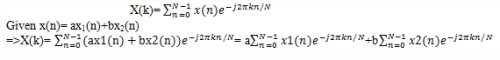=>X(k)= aX1(k)+bX2(k).

## Signals and Systems

32 videos|76 docs|63 tests
 Use Code STAYHOME200 and get INR 200 additional OFF Use Coupon Code
Information about Test: DFT Properties Page
In this test you can find the Exam questions for Test: DFT Properties solved & explained in the simplest way possible. Besides giving Questions and answers for Test: DFT Properties, EduRev gives you an ample number of Online tests for practice

## Signals and Systems

32 videos|76 docs|63 tests